﻿ Getting Started Tutorials - MechDesigner > Tutorial 13: Forces: Introduction  > Step 13.2: Rocker and a Spring

# Step 13.2: Rocker and a Spring

### A Rocker and a Spring

In this topic, we balance the forces that act on the Rocker with a Spring.

Downward Force and Clockwise Moment on the Rocker that result from Gravitational Force

is balanced by ...

An Upward Force and Counter-Clockwise Moment on the Rocker from a Spring

The Rocker is stationary.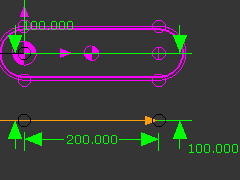A Spring FB exerts a force between two Points in two different Parts. In this case, a Point in the Rocker and a Point in the Base-Part.

STEP 1: Edit the Base-Part to add a Line

 1.Edit the Base-Part 2.Part-Editor: Add a horizontal Line so that its end-Pointsis 100mm below the end of the Rocker. 3.Close the Part-Editor

TOP-TIP: You can use Tools menu > Show other Kinematic and Sketch-Elements to see the Rocker when you edit other Parts in the Part-Editor.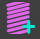STEP 2: Click Force Toolbar > Add Spring FB

To add the Spring FB you must click a Point in two different Parts

 1.Mechanism-Editor: Click the end-Pointof the CAD-Line in the Rocker and not the center-Point of the Arc(+) 2.Mechanism-Editor: Click the end-Point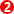of the Line in the Base-Part that is 100mm below the Rocker

The Points become the Anchor-Points for the Spring Fb.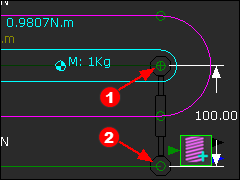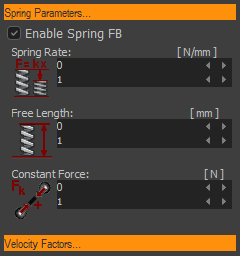STEP 3: Enable the Spring FB

 1.Mechanism-Editor: Double-click the Spring FB in the graphic-area to open the Spring FB dialog-box 2.Spring FB dialog-box: Click the Spring Parameters separator to see its parameters. 3.Spring FB dialog-box: Click the Enable Spring FB check-box The tick should show in the check-box 4.Spring FB dialog-box: Click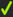to close the Spring FB dialog-box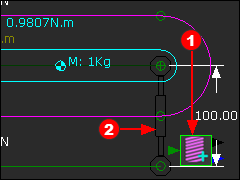The image to the left shows the Spring Function-Blockand the Symbolthat represents the Spring FB.

The Spring FB does not exert a Force, yet. Thus, MechDesigner does not show Force Vecotrs at the Spring's anchor-points.

Constant Force

We can balance the Gravitational Force if we enter a Constant Force in the Spring Force dialog-box.

A positive Constant-Force pulls the Spring's anchor-points. (o>>>+<<<o)

A negative Contact-Force pushes the Spring's anchor-points (o<<<–>>>o)

STEP 4: Edit the Spring FB to enter a Constant-Force= -9.80665N

 1.Mechanism-Editor: Double-click the Spring FB in the graphic-area to open the Spring FB dialog-box 2.Spring FB dialog-box: Click the Spring Parameters separator to see its parameters. 3.Spring FB dialog-box: Constant-Force enter -9.80665 4.Spring FB dialog-box: Clickto close the Spring FB dialog-box

-9.80665N exerts a Force upwards against the Rocker.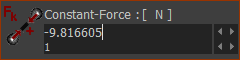-9.8066N
Negative Force pusshes Point apart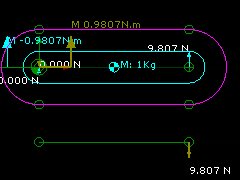Addition of Vertical Forces acting on the Rocker  (upwards +ve).

∑FV=0 : R2(N) -1(kg)*9.807(m/s/s) + 9.807(N) = 0; R2 = 0N

Addition of Horizontal Forces acting on the Rocker (Point 2) : (upwards +ve).

∑FH=0 : R2H(N) = 0;  R2H = 0N

Take Moments about Rotational Center of the Rocker (Counter-clockwise +ve)

∑M1=0 ; M2 + 0.2(m)*9.81(N) - 0.1(m)*9.81(N) = 0 . Thus M2= -0.981Nm.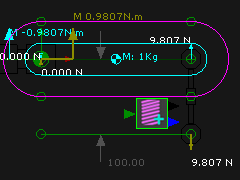Spring-Rate and Free-Length

You can use the Spring-Rate and Free-Length Parameters to obtain the same result.

We will enter a Free-Length and Spring-Rate so that the Spring exerts a force equal and opposite to the Gravitational Force.

Also, edit the Constant Force = 0N again.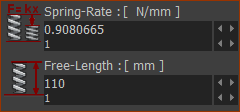When we make the Free-Length = 110mm and the Spring-Rate = 0.980665N/mm, the Spring FB will exert 9.80665N upwards.

Why? The model defines the actual distance between the anchor-points of the Spring FB as 100mm.

Therefore, the Spring FB is deflected by the model by 10mm

Therefore, the Spring FB exerts a Force = 10mm * 0.98066N/mm =  9.81N upwards

Note: The Spring FB has:

Two output-connectors :

Top output-connector = Distance, velocity and acceleration of one anchor-point relative to the other.

Bottom output-connector =Total Force, X-Force and Y-Force (relative to the coordinate axes of the Mechanism-Plane).

One input Connector :

Optionally, a Force Function at its input-connector from a Math FB.

The Output Data-Type of the Math FB must be set to Force.